# NCERT Solutions for Class 8 Maths Exercise 5.3## myCBSEguide App

CBSE, NCERT, JEE Main, NEET-UG, NDA, Exam Papers, Question Bank, NCERT Solutions, Exemplars, Revision Notes, Free Videos, MCQ Tests & more.

NCERT Solutions for Class 8 Maths Exercise 5.3 Class 8 Maths book solutions are available in PDF format for free download. These ncert book chapter wise questions and answers are very helpful for CBSE exam. CBSE recommends NCERT books and most of the questions in CBSE exam are asked from NCERT text books. Class 8 Maths chapter wise NCERT solution for Maths Book all the chapters can be downloaded from our website and myCBSEguide mobile app for free.

NCERT solutions for Class 8 Maths Data Handling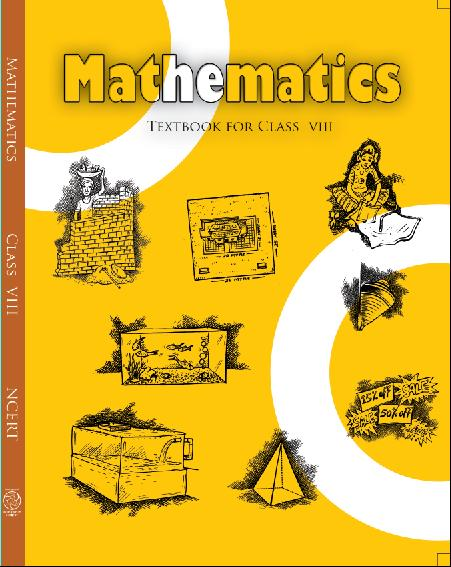## NCERT Solutions for Class 8 Maths Data Handling

###### 1. List the outcomes you can see in these experiments.

(a) Spinning a wheel  (b) Tossing two coins together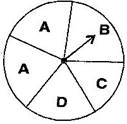Ans. (a) There are four letters A, B, C and D in a spinning wheel. So there are 4 outcomes.

(b) When two coins are tossed together. There are four possible outcomes HH, HT, TH, TT.

(Here HT means head on first coin and tail on second coin and so on.)

NCERT Solutions for Class 8 Maths Exercise 5.3

###### 2. When a die is thrown, list the outcomes of an event of getting:

(i) (a) a prime number

(b) not a prime number

(ii) (a) a number greater than 5

(b) a number not greater than 5

Ans. (i) (a) Outcomes of event of getting a prime number are 2, 3 and 5.

(b) Outcomes of event of not getting a prime number are 1, 4 and 6.

(ii) (a) Outcomes of event of getting a number greater than 5 is 6.

(b) Outcomes of event of not getting a number greater than 5 are 1, 2, 3, 4 and 5.

NCERT Solutions for Class 8 Maths Exercise 5.3

###### 3. Find the: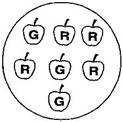(a) Probability of the pointer stopping on D in (Question 1 (a)).

(b) Probability of getting an ace from a well shuffled deck of 52 playing cards.

(c) Probability of getting a red apple. (See figure alongside)

Ans. (a) In a spinning wheel, there are five pointers A, A, B, C, D. So there

are five outcomes. Pointer stops at D which is one outcome.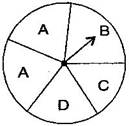So the probability of the pointer stopping on D =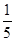(b) There are 4 aces in a deck of 52 playing cards. So, there are four events of getting an ace.

So, probability of getting an ace =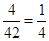(c) Total number of apples = 7

Number of red apples = 4

Probability of getting red apple =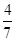NCERT Solutions for Class 8 Maths Exercise 5.3

###### 4. Numbers 1 to 10 are written on ten separate slips (one number on one slip), kept in a box and mixed well. One slip is chosen from the box without looking into it. What is the probability of:

(i) getting a number 6.

(ii) getting a number less than 6.

(iii) getting a number greater than 6.

(iv) getting a 1-digit number.

Ans. (i) Outcome of getting a number 6 from ten separate slips is one.

Therefore, probability of getting a number 6 =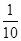(ii) Numbers less than 6 are 1, 2, 3, 4 and 5 which are five. So there are 5 outcomes.

Therefore, probability of getting a number less than 6 =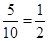(iii) Number greater than 6 out of ten that are 7, 8, 9, 10.So there are 4 possible outcomes.

Therefore, probability of getting a number greater than 6 =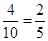(iv) One digit numbers are 1, 2, 3, 4, 5, 6, 7, 8, 9 out of ten.

Therefore, probability of getting a 1-digit number =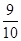NCERT Solutions for Class 8 Maths Exercise 5.3

###### 5. If you have a spinning wheel with 3 green sectors, 1 blue sector and 1 red sector, what is the probability of getting a green sector? What is the probability of getting a none-blue sector?

Ans. There are five sectors. Three sectors are green out of five sectors.

Therefore, probability of getting a green sector =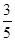There is one blue sector out of five sectors.

Non-blue sectors = 5 – 1 = 4 sectors

Therefore, probability of getting a non-blue sector =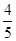NCERT Solutions for Class 8 Maths Exercise 5.3

###### 6. Find the probability of the events given in Question 2.

Ans. When a die is thrown, there are total six outcomes, i.e., 1, 2, 3, 4, 5 and 6.

(i) (a) 2, 3, 5 are prime numbers. So there are 3 outcomes out of 6.

Therefore, probability of getting a prime number =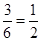(b) 1, 4, 6 are not the prime numbers. So there are 3 outcomes out of 6.

Therefore, probability of getting a prime number =(ii) (a) Only 6 is greater than 5. So there is one outcome out of 6.

Therefore, probability of getting a number greater than 5 =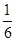(b) Numbers not greater than 5 are 1, 2, 3, 4 and 5. So there are 5 outcomes out of 6.

Therefore, probability of not getting a number greater than 5 =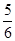## NCERT Solutions for Class 8 Maths Exercise 5.3

NCERT Solutions Class 8 Mathematics PDF (Download) Free from myCBSEguide app and myCBSEguide website. Ncert solution class 8 Mathematics includes text book solutions from Class 8 Maths Book . NCERT Solutions for CBSE Class 8 Maths have total 16 chapters. 8 Maths NCERT Solutions in PDF for free Download on our website. Ncert class 8 solutions PDF and Maths ncert class 8 PDF solutions with latest modifications and as per the latest CBSE syllabus are only available in myCBSEguide.

## CBSE app for Class 8

To download NCERT Solutions for class 8 Social Science, Computer Science, Home Science,Hindi ,English, Maths Science do check myCBSEguide app or website. myCBSEguide provides sample papers with solution, test papers for chapter-wise practice, NCERT solutions, NCERT Exemplar solutions, quick revision notes for ready reference, CBSE guess papers and CBSE important question papers. Sample Paper all are made available through the best app for CBSE students and myCBSEguide website.### 5 thoughts on “NCERT Solutions for Class 8 Maths Exercise 5.3”

1. This website is good